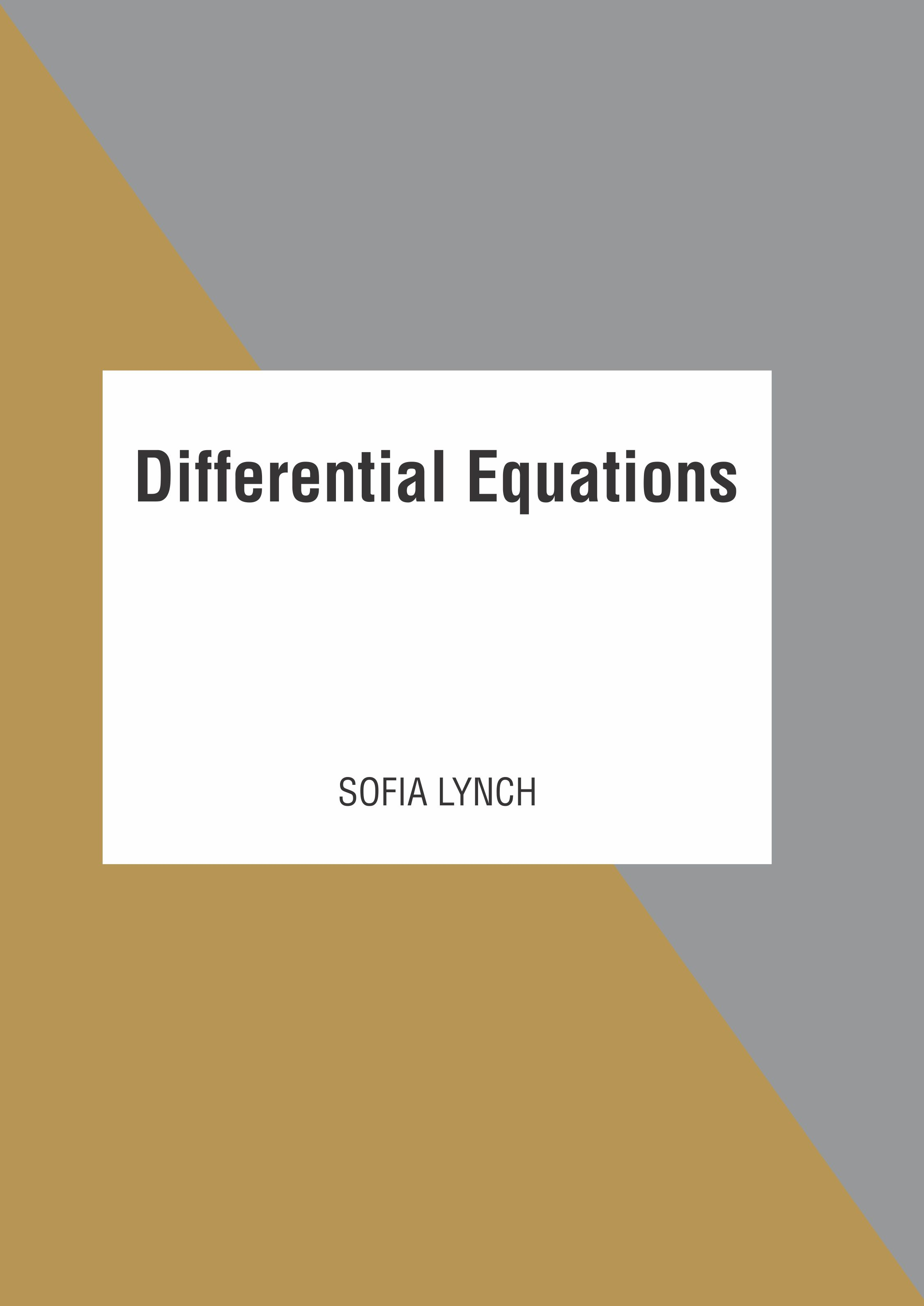# books

BROWSE BY

#### Author

Results 1 - 2 of 2Differential Equations Author : Sofia Lynch Subject : Botany A mathematical equation which relates some function with its derivatives is known as a differential equation. While applying a differential equation, the physical quantities are represented by functions Read MoreDifferential Equations Author : Sofia Lynch Subject : Botany A mathematical equation which relates some function with its derivatives is known as a differential equation. While applying a differential equation, the physical quantities are represented by functions Read More
Results 1 - 2 of 2